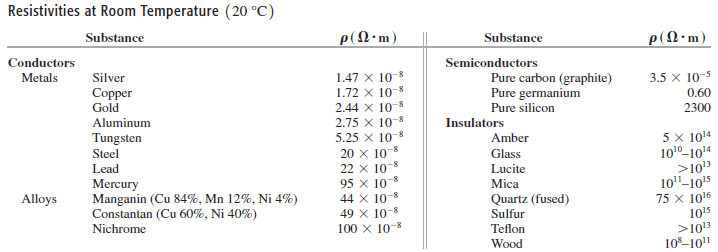# Problem: Pure silicon contains approximately 1.0x 1016 free electrons per cubic meter.(a) Referring to the table above, calculate the mean free time ? for silicon at room temperature.(b) Your answer in part (a) is much greater than the mean free time for copper. Why, then, does pure silicon have such a high resistivity compared to copper?

###### FREE Expert Solution

The mean free time:

$\overline{){{\mathbf{T}}}_{{\mathbf{\lambda }}}{\mathbf{=}}\frac{\mathbf{m}}{\mathbf{n}{\mathbf{e}}^{\mathbf{2}}\mathbf{\rho }}}$

(a)

For silicon, m = 9.1 × 10-31, n = 1.0 × 1016 electrons/m3, e = 1.6 × 10-19C and ρ = 2300Ω•m.

97% (256 ratings)###### Problem Details

Pure silicon contains approximately 1.0x 1016 free electrons per cubic meter.(a) Referring to the table above, calculate the mean free time ? for silicon at room temperature.

(b) Your answer in part (a) is much greater than the mean free time for copper. Why, then, does pure silicon have such a high resistivity compared to copper?

Frequently Asked Questions

What scientific concept do you need to know in order to solve this problem?

Our tutors have indicated that to solve this problem you will need to apply the Nuclear Physics concept. You can view video lessons to learn Nuclear Physics. Or if you need more Nuclear Physics practice, you can also practice Nuclear Physics practice problems.